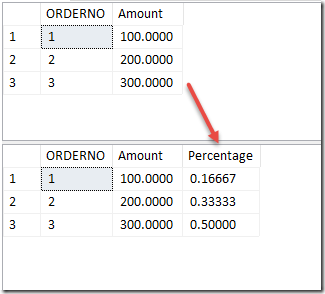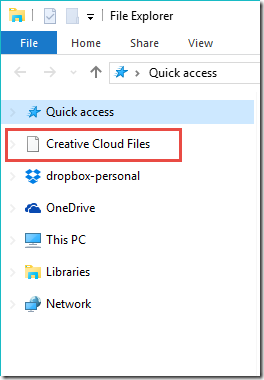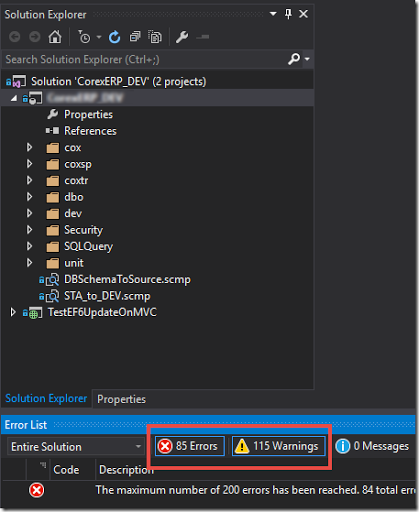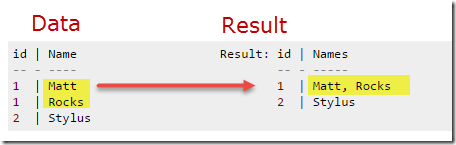## 發表文章

### T-SQL 計算佔全部結果的比率### Remove Creative Cloud Files### T-SQL Update with join table

(A) T-SQL 更新資料的最基本語法格式 UPDATE dbo.Table1 SET CalculatedColumn='New Value' WHERE t1.BatchNo = '110'; (B) 當遇到更新的值需要查詢來取得，原本我只會這樣： UPDATE dbo.Table1 SET CalculatedColumn= (SELECT [Calculated Column] FROM dbo.Table2 WHERE dbo.Table1.CommonField=dbo.Table2.[Common Field] ) WHERE t1.BatchNo = '110'; (C) 前一陣子，偶然+赫然發現原來可以先 Join 再更新 UPDATE t1 SET t1.CalculatedColumn = t2.[Calculated Column] FROM dbo.Table1 AS t1 INNER JOIN dbo.Table2 AS t2 ON t1.CommonField = t2.[Common Field] WHERE t1.BatchNo = '110'; (D) 另一個採用 CTE (COMMON TABLE EXPRESSION) 的花式應用 ;WITH t2 AS ( SELECT [key], CalculatedColumn = SUM(some_column) FROM dbo.table2 GROUP BY [key] ) UPDATE t1 SET t1.CalculatedColumn = t2.CalculatedColumn FROM dbo.table1 AS t1 INNER JOIN t2 ON t1.[key] = t2.[key]; 使用心得 當遇到需要先 JOIN 兩個資料表再做更新時，上述 (B) (C) (D) 三種語法，在實際試過幾種不同狀況，發現針對效能考量上並無一種絕對的作法，得視資料量大小與串聯邏輯去設計與嘗試找到最佳解。 參考文章 Update a table using JOIN in SQL Server? – stackov

### 資料庫專案加入參考### 組合多筆資料為一個字串